# Pease answer questions 4 and 5! Thank you so much! 4. Connect the 2nd resistor in...

Pease answer questions 4 and 5! Thank you so much!

4. Connect the 2nd resistor in parallel with the 1st resistor and connect that combination to the battery.

Measure the following:

The current passing through the R1, I1 = ___0.0058____ A

The current passing through the R2, I2 = ___0.0031____ A

The current supplied by the battery, I = ___0.0092____ A

Calculate I1 + I2 = ____________ A

Is the following statement true? I = I1 + I2 _____Y or N______

Calculate the energy delivered by the battery

= V I = ____________ x ____________

= ___________ Watts

Why is the power delivered by the battery greater than in Step 1?

__________________________________________________________

__________________________________________________________

5. Disconnect the battery from the circuit (but leave the two resistors connected in parallel).

Use the Multimeter to measure the combined resistance of the two resistors connected in parallel:

Measure the equivalent resistance, R = ___680___ Ohms

Calculate 1/R, which equals to:

1/R = 1/R1 + 1/R2 = 1/( ) + 1/ ( )

= __________ + __________

= ____________

Calculate R = ____________ Ohms

Is the calculated R and measured R approximately equal to each other?

____________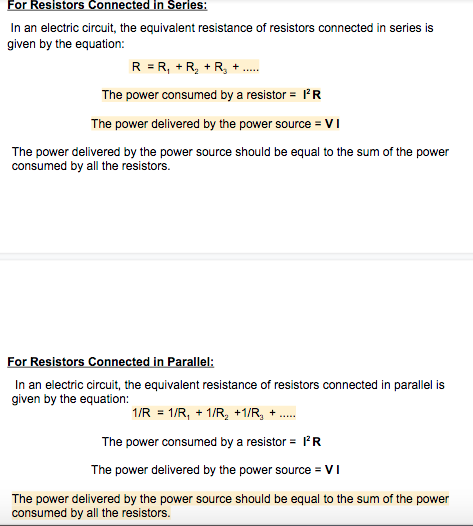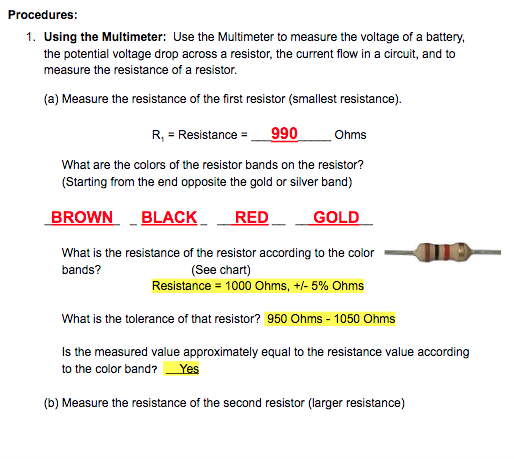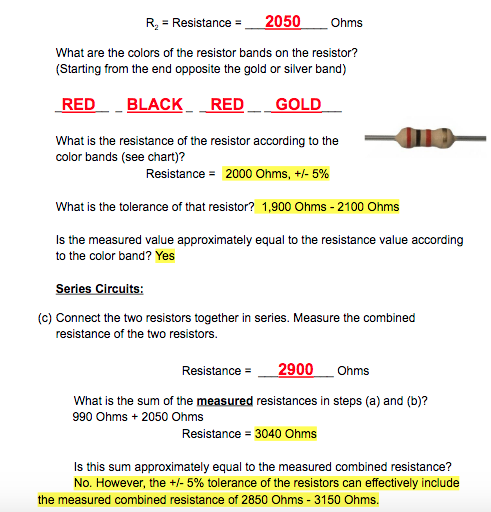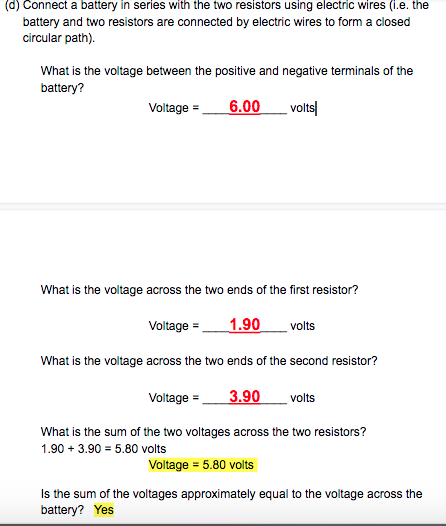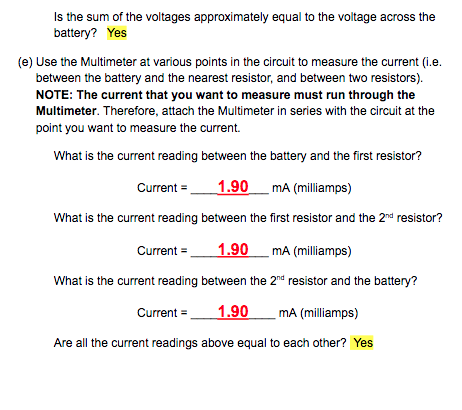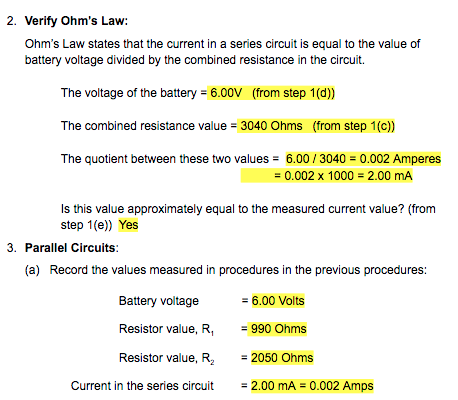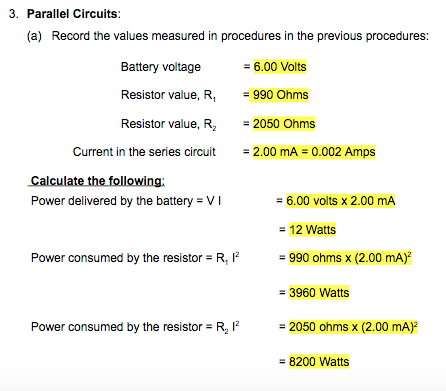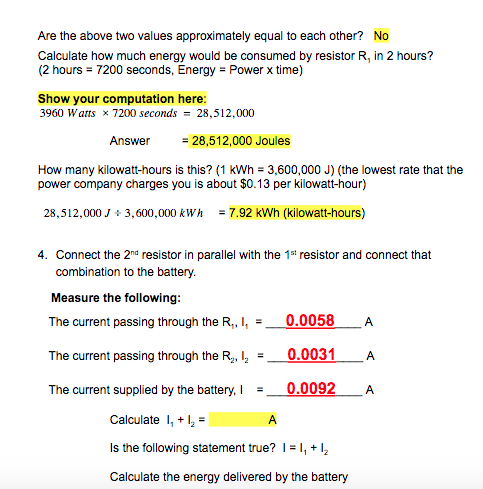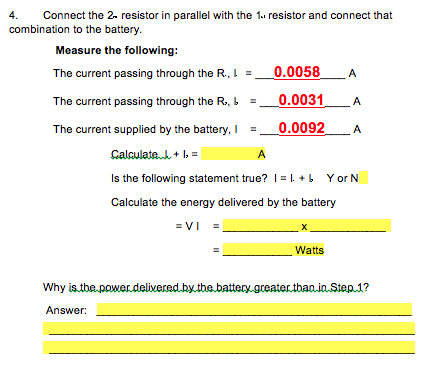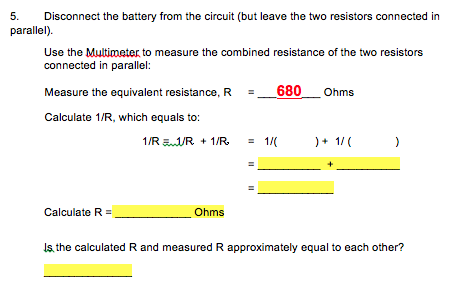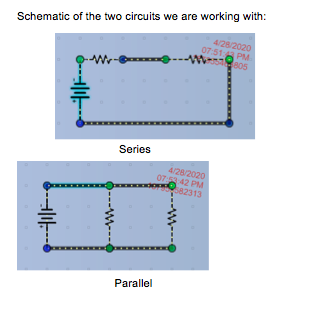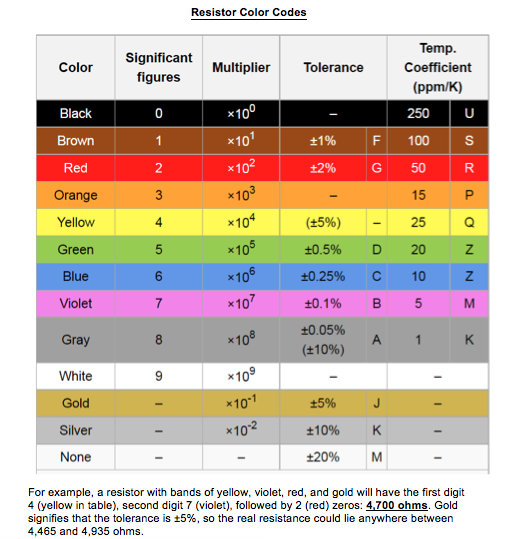For Resistors Connected in Series: In an electric circuit, the equivalent resistance of resistors connected in series is given by the equation: R=R, + R, + R. +..... The power consumed by a resistor = 1R The power delivered by the power source = VI The power delivered by the power source should be equal to the sum of the power consumed by all the resistors. For Resistors Connected in Parallel: In an electric circuit, the equivalent resistance of resistors connected in parallel is given by the equation: 1/R = 1/R, + 1/R, +1/R, + The power consumed by a resistor = 'R The power delivered by the power source = VI The power delivered by the power source should be equal to the sum of the power consumed by all the resistors.
Procedures: 1. Using the Multimeter: Use the Multimeter to measure the voltage of a battery, the potential voltage drop across a resistor, the current flow in a circuit, and to measure the resistance of a resistor. (a) Measure the resistance of the first resistor (smallest resistance). R, Resistance = 990 Ohms What are the colors of the resistor bands on the resistor? (Starting from the end opposite the gold or silver band) BROWN BLACK RED GOLD What is the resistance of the resistor according to the color bands? (See chart) Resistance = 1000 Ohms, +/- 5% Ohms What is the tolerance of that resistor? 950 Ohms - 1050 Ohms Is the measured value approximately equal to the resistance value according to the color band? Yes (b) Measure the resistance of the second resistor (larger resistance)
R, = Resistance = 2050 Ohms What are the colors of the resistor bands on the resistor? (Starting from the end opposite the gold or silver band) RED BLACK RED GOLD What is the resistance of the resistor according to the color bands (see chart)? Resistance = 2000 Ohms, +/- 5% What is the tolerance of that resistor? 1,900 Ohms - 2100 Ohms Is the measured value approximately equal to the resistance value according to the color band? Yes Series Circuits: (c) Connect the two resistors together in series. Measure the combined resistance of the two resistors. Resistance 2900 Ohms What is the sum of the measured resistances in steps (a) and (b)? 990 Ohms + 2050 Ohms Resistance = 3040 Ohms Is this sum approximately equal to the measured combined resistance? No. However, the +/- 5% tolerance of the resistors can effectively include the measured combined resistance of 2850 Ohms - 3150 Ohms.
(c) Connect a battery in series with the two resistors using electric wires (.e. the battery and two resistors are connected by electric wires to form a closed circular path). What is the voltage between the positive and negative terminals of the battery? Voltage = _ 6.00 volts What is the voltage across the two ends of the first resistor? Voltage = 1.90 volts What is the voltage across the two ends of the second resistor? Voltage = 3.90 volts What is the sum of the two voltages across the two resistors? 1.90 + 3.90 = 5.80 volts Voltage = 5.80 volts Is the sum of the voltages approximately equal to the voltage across the battery? Yes
Is the sum of the voltages approximately equal to the voltage across the battery? Yes (e) Use the Multimeter at various points in the circuit to measure the current (i.e. between the battery and the nearest resistor, and between two resistors). NOTE: The current that you want to measure must run through the Multimeter. Therefore, attach the Multimeter in series with the circuit at the point you want to measure the current. What is the current reading between the battery and the first resistor? Current = 1.90 mA (milliamps) What is the current reading between the first resistor and the 2nd resistor? Current = 1.90 mA (milliamps) What is the current reading between the 2nd resistor and the battery? Current = 1.90 mA (milliamps) Are all the current readings above equal to each other? Yes
2. Verify Ohm's Law: Ohm's Law states that the current in a series circuit is equal to the value of battery voltage divided by the combined resistance in the circuit The voltage of the battery = 6.00V (from step 1(d)) The combined resistance value = 3040 Ohms (from step 1(C)) The quotient between these two values = 6.00 / 3040 = 0.002 Amperes = 0.002 x 1000 = 2.00 mA Is this value approximately equal to the measured current value? (from step 1(e)) Yes 3. Parallel Circuits: (a) Record the values measured in procedures in the previous procedures: Battery voltage Resistor value, R Resistor value, R2 = 6.00 Volts = 990 Ohms = 2050 Ohms = 2.00 mA = 0.002 Amps Current in the series circuit
3. Parallel Circuits: (a) Record the values measured in procedures in the previous procedures: = 6.00 Volts Battery voltage Resistor value, R = 990 Ohms = 2050 Ohms = 2.00 mA = 0.002 Amps Resistor value, R Current in the series circuit Calculate the following: Power delivered by the battery = VI = 6.00 volts x 2.00 mA = 12 Watts Power consumed by the resistor = R, 12 = 990 ohms x (2.00 mA) = 3960 Watts Power consumed by the resistor = R, 12 = 2050 ohms x (2.00 mA) = 8200 Watts
Are the above two values approximately equal to each other? No Calculate how much energy would be consumed by resistor R, in 2 hours? (2 hours = 7200 seconds, Energy = Power x time) Show your computation here: 3960 Watts x 7200 seconds = 28,512,000 Answer = 28,512,000 Joules How many kilowatt-hours is this? (1 kWh = 3,600,000 J) (the lowest rate that the power company charges you is about \$0.13 per kilowatt-hour) 28,512,000 + 3,600,000 kWh = 7.92 kWh (kilowatt-hours) 4. Connect the 2nd resistor in parallel with the 1s resistor and connect that combination to the battery. Measure the following: The current passing through the R., I, = 0.0058 A The current passing through the Ry, 12 = 0.0031 A The current supplied by the battery, I 0.0092 A A Calculate 1, + 12 = Is the following statement true? I = 1, + 12 Calculate the energy delivered by the battery
4. Connect the 2. resistor in parallel with the 1.. resistor and connect that combination to the battery. Measure the following: The current passing through the R., 1 = 0.0058 A The current passing through the R., b = 0.0031 A The current supplied by the battery, I 0.0092 A Calculated + 1 = A Is the following statement true? | = 1. + b YorN Calculate the energy delivered by the battery =VI х Watts Why is the power delivered by the battery greater than in Step.1? Answer:
5. Disconnect the battery from the circuit (but leave the two resistors connected in parallel). Use the Multimeter to measure the combined resistance of the two resistors connected in parallel: Measure the equivalent resistance, R 680 Ohms Calculate 1/R, which equals to: 1/R=1/R + 1/R = 1/ ) + 11 ( 11 ) Calculate R = Ohms Is the calculated R and measured R approximately equal to each other?
Schematic of the two circuits we are working with: 4/28/2020 07:51 PM 805 Series 4/28/2020 OT 42 PM 882313 Parallel
Resistor Color Codes Color Significant figures Multiplier Tolerance 0 Temp. Coefficient (ppm/K) 250 U 100 s 50 R 15 Р +1% F 2 +2% G *10° *10 x10² *103 x104 *105 Black Brown Red Orange Yellow Green Blue Violet 3 4 - 25 Q (15%) +0.5% 5 D 20 z 6 x106 +0.25% C N 10 5 *10? 0.1% B M Gray 8 *10 10.05% (+10%) A 1 K 9 *10° J 1 White Gold Silver None *101 *102 +5% +10% +20% к M For example, a resistor with bands of yellow, violet, red, and gold will have the first digit 4 (yellow in table), second digit 7 (violet), followed by 2 (red) zeros: 4,700 ohms. Gold signifies that the tolerance is 15%, so the real resistance could lie anywhere between 4,465 and 4,935 ohms.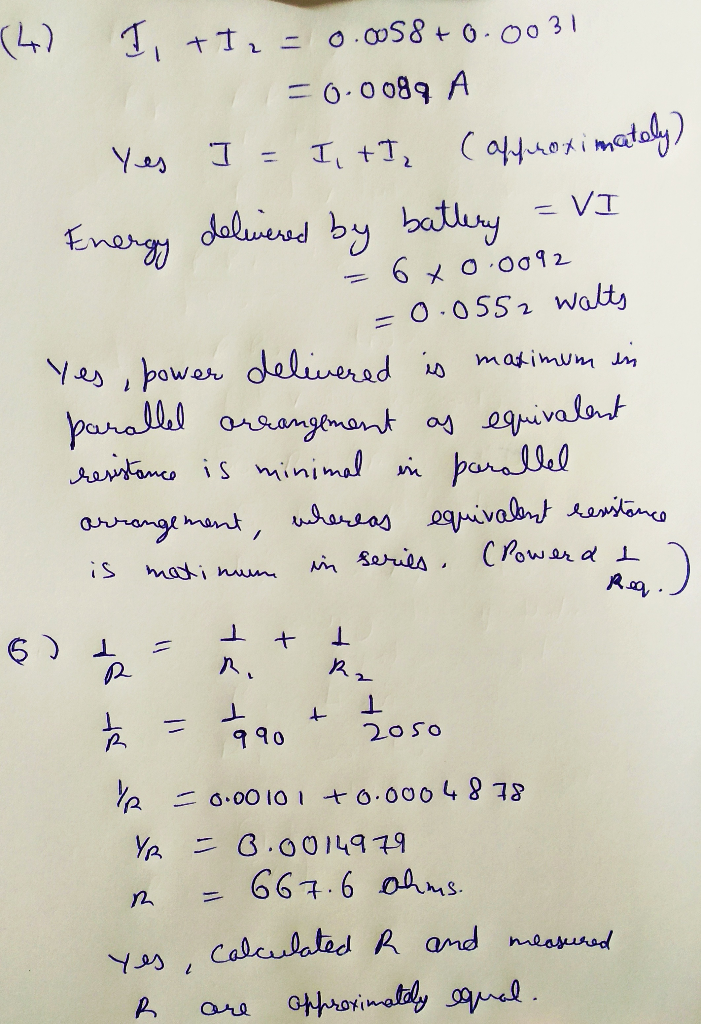##### Add Answer of: Pease answer questions 4 and 5! Thank you so much! 4. Connect the 2nd resistor in...
Similar Homework Help Questions
• ### Answer 4 & 5 please! thank you! R values = Resistor value, R1 = 990 Ohms...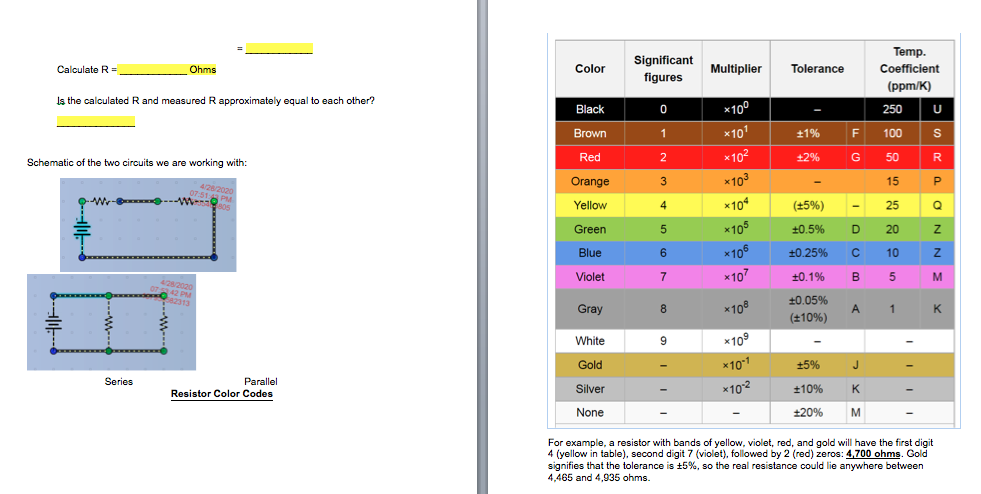Answer 4 & 5 please! thank you! R values = Resistor value, R1 = 990 Ohms Resistor value, R2 = 2050 Ohms 4. Connect the 2nd resistor in parallel with the 1st resistor and connect that combination to the battery.          Measure the following:               The current passing through the R1, I1 = ___0.0058____ A               The current passing through the R2, I2 = ___0.0031____ A               The current supplied by the battery, I = ___0.0092____ A              Calculate I1 + I2 =...

• ### R1 R3 R2 R4 W The circuit in the diagram contains one battery and four resistors,...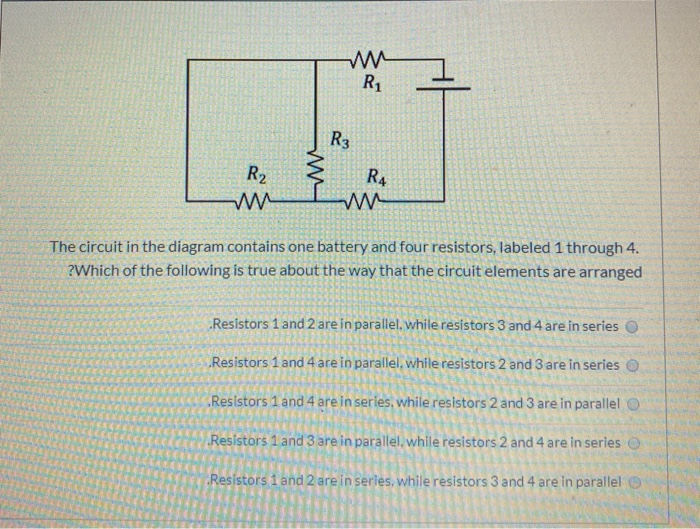R1 R3 R2 R4 W The circuit in the diagram contains one battery and four resistors, labeled 1 through 4. ?Which of the following is true about the way that the circuit elements are arranged Resistors 1 and 2 are in parallel while resistors 3 and 4 are in series Resistors 1 and 4 are in parallel while resistors 2 and 3 are in series Resistors 1 and 4 are in series, while resistors 2 and 3 are in parallel...

• ### ELEC. eng

A 50 Ohm resistor is connected in parallel with a 100 Ohm resistor. Which of the following statements are true ?1. The total resistance is less than 50 Ohms2. The two resistors have the same voltage across them.3. The two resistors are connected back to back4. All of these statements are true.A circuit has a Thevenin equivalent circuit with Vth = 12 Volts and Rth = 50 Ohms. The load resistance that gives maximum power transfer is :1. 100 Ohms2....

• ### Experiment RESISTANCE IN COMBINATION PURPOSE: To study series, parallel, and series.parallel combinations of resistors. EQUIPMENTThe experiment...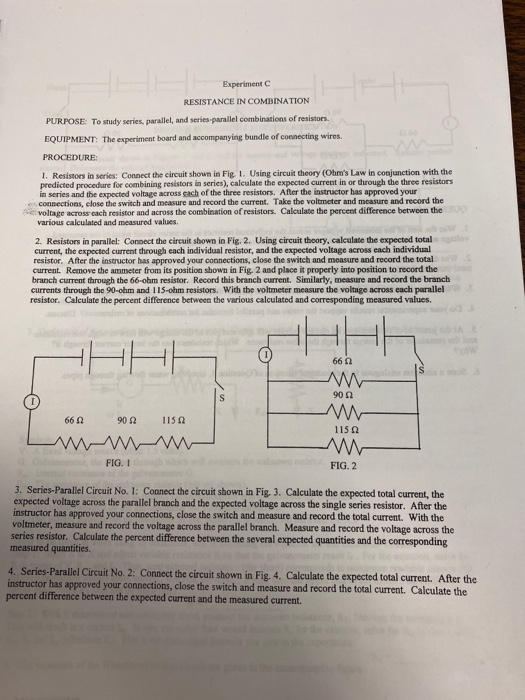Experiment RESISTANCE IN COMBINATION PURPOSE: To study series, parallel, and series.parallel combinations of resistors. EQUIPMENTThe experiment board and accompanying bundle of connecting wires. PROCEDURE: 1. Resistors in series: Connect the circuit shown in Fig. 1. Using circuit theory (Ohms Law in conjunction with the predicted procedure for combining resistors in series), calculate the expected current in or through the three resistors in series and the expected voltage across each of the three resistors. After the instructor has approved your connections,...

• ### IMMEDIATE LIFESAVER FOR CORRECT ANSWERS AND GOOD EXPLANATIONS!! Five identical resistors, each...

1)a) Five identical resistors, each of resistance R, are connected in series to a battery, and a current of 8.27 A flows in the circuit. Now, another resistor ofresistance R is added to the circuit, in series with the rest of the resistors. Find the new current in the circuit._______ Ab) Five identical resistors, each of resistance R, are connected in parallel to a battery, and a current of 8.27 A flows in the circuit. Now, another resistor ofresistance R...

• ### resistors in series and in parallel

1. the terminal voltage of a battery is found to be 4.2 volts. two resistors of 2 and 4 ohms are connected in series to the battery. A single resistor of unknownresistance is connected in parallel to the two resistors. A current of 1.4 amp is registered in the ammeter of the unknown resistor. what is the current in 2 ohmsresistor? what is the voltage of the 4 ohm resistor?

• ### Pre-lab EM-5 Ohm's Law and Kirchhoff's Rules Ohm's Law The resistance R of a device can...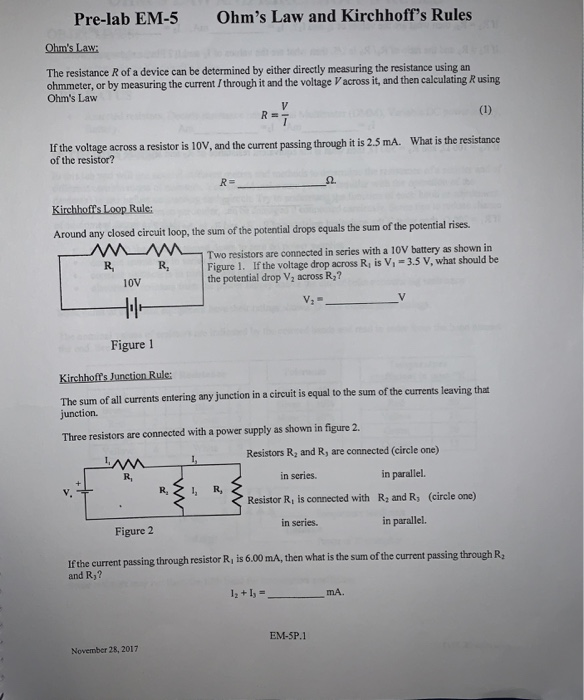Pre-lab EM-5 Ohm's Law and Kirchhoff's Rules Ohm's Law The resistance R of a device can be determined by either directly measuring the resistance using an ohmmeter, or by measuring the current I through it and the voltage Vacross it, and then calculating R using Ohm's Law V R= (1) If the voltage across a resistor is 10V, and the current passing through it is 2.5 mA. of the resistor? What is the resistance R= Ω. Kirchhoffs Loop Rule Around...

• ### 14-5 points A 3. Q resistor is connected in parallel with a 6. Q resistor. This...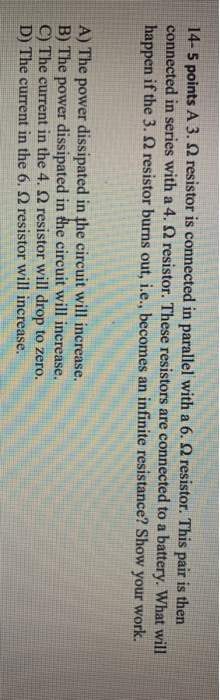14-5 points A 3. Q resistor is connected in parallel with a 6. Q resistor. This pair is then connected in series with a 4. 2 resistor. These resistors are connected to a battery. What will happen if the 3. resistor burns out, i.e., becomes an infinite resistance? Show your work. A) The power dissipated in the circuit will increase. B) The power dissipated in the circuit will increase. C) The current in the 4.12 resistor will drop to zero....

• ### A 1.40 kΩ resistor is connected in series with a 0.800 kΩ resistor. This pair of...

A 1.40 kΩ resistor is connected in series with a 0.800 kΩ resistor. This pair of resistors is connected in parallel with a 7.00 kΩ resistor. That combination is then connected in series with a 0.700 kΩ resistor and a 9.0-V ideal battery (no internal resistance of course). A) sketch this circuit B) Determine the total equivalent resistance of this circuit's arrangement C) Determine the amount of current that flows through the battery D) Determine the voltage drop across the...4. complete the following statement: A simple series circuitcontains a resistance R and an ideal battery. If a second resistoris connected in parallel with R,A. the voltage across R will decreaseB. the current through R will decreaseC. the total current through the battery will increaseD. the rate of energy dissipation in R will increaseE. the equivalent resistance of the circuit willincrease5. two resistors are arranged in acircuit that carriesa total current of 15A as shown in the figure. what is...